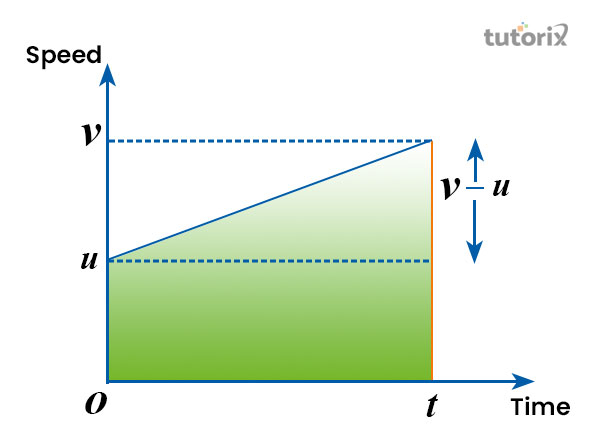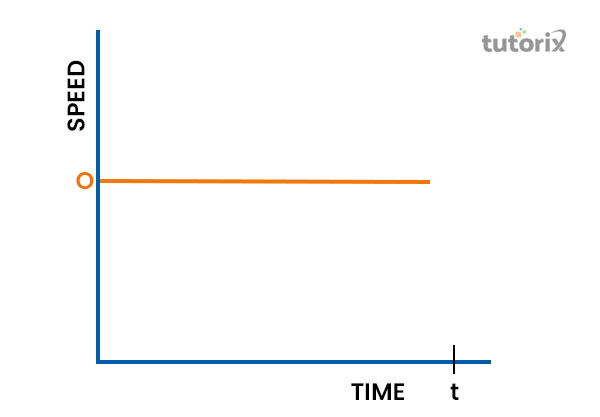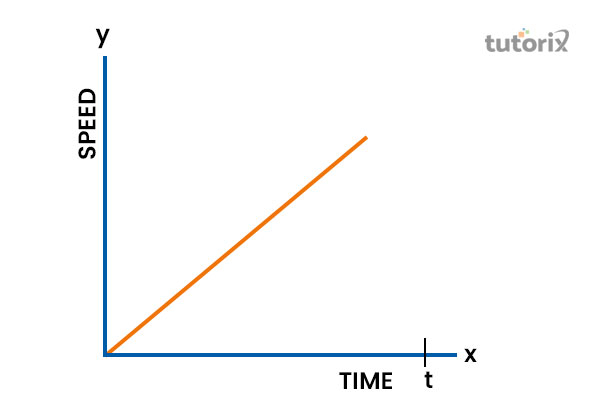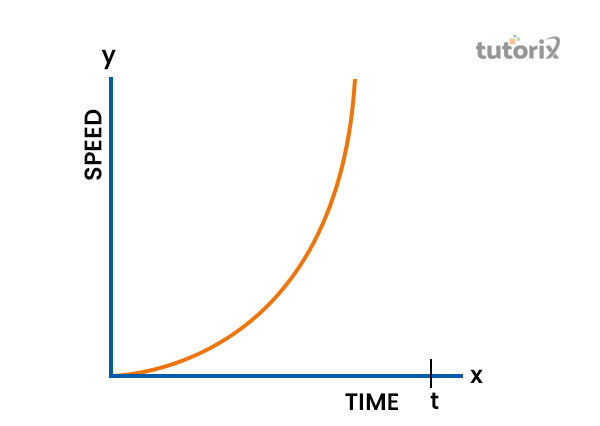# Speed Time Graphs

## Introduction

The speed-time graphs are commonly used while showcasing the basic difference between time and velocity in real-time. Whenever speed is constant with zero acceleration, a speed-time graph is drawn in order to outline the difference between speed and velocity there. Under different circumstances, where speed and velocity are present, the speed-time graphs intervene to help in understanding the difference between those two in real-time.

## Definition of Speed-Time Graphs

The speed-time graphs are extremely useful graphs used in determining an object's movement at a certain point in time. It allows people to see, at what speed an object is moving in real-time and what is the current velocity of the object while moving.

In a speed-time graph, speed is always plotted on the vertical axis and time is plotted on the horizontal axis (Bbc, 2022). Both the axis in the graph represents the motion of an object that is accelerating from a certain speed at the time.Figure 1: Speed-time graph

In a speed-time graph, time is generally 0, u to a speed v at a certain point of time. The gradient within a speed-time graph represents the acceleration of an object where the acceleration is constant. The other areas of a speed-time graph generally outline uniform acceleration and constant velocity at a certain point in time (Ncl, 2022).

The gradient of the line within a speed-time graph can be calculated by dividing the change of velocity with time while drawing the graph.

## Types of Speed-Time Graphs

### With Constant Speed

Within a speed-time graph where the speed is constant, the Y axis denotes the speed and the X axis denotes the time. According to these types of speed-time graphs, speed is always constant throughout the time till a certain point of the interval.Figure 2: Speed-time graph with constant speed

Within this type of speed-time graph, it does not matter how much time is changing but the speed will be constant throughout the course of time. So, according to this scenario, when speed is constant, it does not consider the elapsed time while flowing (Li et al. 2019).

• For example, if the acceleration of an object is 0 and the speed is constant (5 m/s) then the equation stands 5 m/s at t = 0. Here, the speed of the object is going to remain constant throughout the course of time.

### With Constant Acceleration

When an object's acceleration is constant and the initial speed of the object is 0, the speed of the object is going to increase linearly as shown in the speed-time graph below. In this type, the equation remains v = u + at. Since here u is equal to 0, the v here will automatically be equal with the acceleration (Geogebra, 2022).

So the equation here is v = at when the acceleration is constant.Figure 3: Speed-time graph with constant acceleration

According to the rules of this type of speed-time graph, the speed of the object will linearly increase concerning time. Here, the slope of the graph is going to provide the object with the magnitude of acceleration (Harrod, 2018). For example, if the acceleration of an object is constant (denoted by K) with an initial speed of 0, the speed of the object will increase or decrease linearly. Here, the slope of the graph is going to provide the magnitude of acceleration of the object.

### With Increasing Acceleration

According to this type of speed-time graph, if the acceleration of an object increases with time, the graph will be curved. The equation for the graph here stands at v = u + at. Since the value of u here is 0, the value of v here will be equal to at. So, the equation here is going to be v = at.Figure 4: Speed-time graph with increasing acceleration

In this speed-time graph, the acceleration of the object is a function of time which eventually makes the graph curve. For example, if the acceleration of an object appears as a function of time and the speed is 0, then the speed-time graph will automatically be curved. Here, the slope of the graph is going to give away the magnitude of acceleration at that time.

## Applications of Speed-Time Graphs

The speed-time graph is an extremely useful component for describing the movement of an object under different circumstances. By using data from a speed-time graph one can easily calculate the acceleration of a moving object in real-time. It outlines the change in speed along with the change in time, which makes it useful in terms of calculating the acceleration of a moving object successfully (Bbc, 2022).

The speed-time graphs can also be used while calculating the distance travelled by an object under different circumstances. Within a speed-time graph, an area is particularly segmented for outlining how much distance a moving object has covered within a certain frame of time.

In addition, this graph is also useful in terms of representing the constant speed of an object. In a speed-time graph, a horizontal line is used to represent the acceleration of an object which eventually helps in outlining the constant speed properly (Ncl, 2022). The speed-time graphs also represent the motion of a moving object to some extent. The motion of an object is generally referred to as the deceleration of an object which can be easily outlined by the speed-time graphs.

## Conclusion

The speed-time graph is essential for showcasing the difference between speed and time when an object is moving. The graph considers the constant speed, constant velocity and uniform acceleration of a moving object in terms of outlining how the speed and time of an object will relate in real-time. The horizontal presentation of the graph is useful in terms of outlining the constant speed along with the deceleration of an object that is moving.

## References

### Journals

Harrod, S. (2018). Construction of Discrete Time Graphs from Real Valued Railway Line Data. In New Trends in Emerging Complex Real Life Problems (pp. 293-302). Springer, Cham. Retrieved from: https://core.ac.uk

Li, J., Han, Z., Cheng, H., Su, J., Wang, P., Zhang, J., & Pan, L. (2019, July). Predicting path failure in time-evolving graphs. In Proceedings of the 25th ACM SIGKDD International Conference on Knowledge Discovery & Data Mining (pp. 1279-1289). Retrieved from: https://arxiv.org/pdf/1905.03994

## Websites

Bbc, (2022). Speed-time graphs. Retrieved from: https://www.bbc.co.uk [Retrieved on 15th June 2022]

Geogebra, (2022). Cases of speed-time graphs. Retrieved from: https://www.geogebra.org [Retrieved on 15th June 2022]

Ncl, (2022). Speed-time graphs. Retrieved from: https://www.ncl.ac.uk [Retrieved on 15th June 2022]

Updated on: 13-Oct-2022

1K+ Views